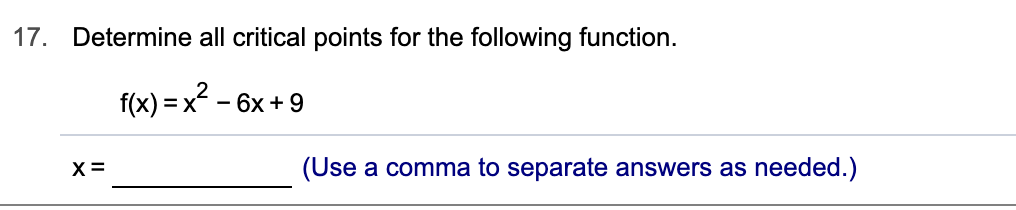# 17.Determine all critical points for the following function.f(x) x6x+9(Use a comma to separate answers as needed.)х3_

Question
9 views

Can you help me step by step?help_outlineImage Transcriptionclose17. Determine all critical points for the following function. f(x) x6x+9 (Use a comma to separate answers as needed.) х3 _ fullscreen
check_circle

Step 1

Critical points of a function f are the points in the domain of f where the derivative is either zero or is not defined.

To find the critical points of a given function, we differentiate the function and set the derivative equal to zero, this (usually) gives us x-values where the derivative is zero and in addition to this we also look for x-vales at which derivative may not be defined.

All these x-values found form the set of critical points for the given function.

We start by differentiation the given function as shown:

Step 2

Next we set the derivative found equal to zero as shown:

Step 3

Observe that 2x-6 being a linear function is defined for all values of x.

Thus, since we have found only one x-value ...

### Want to see the full answer?

See Solution

#### Want to see this answer and more?

Solutions are written by subject experts who are available 24/7. Questions are typically answered within 1 hour.*

See Solution
*Response times may vary by subject and question.
Tagged in

### Calculus